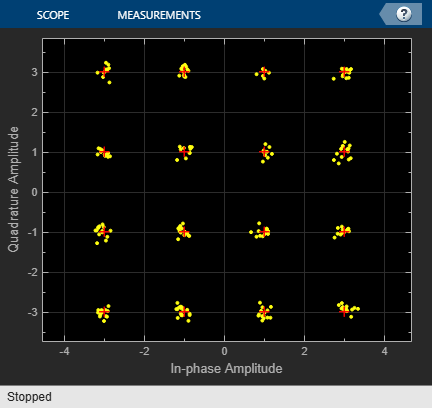## Amplitude Modulation Examples

These examples demonstrate amplitude modulation (AM) techniques.

### Compute Symbol Error Rate

In this example, you generate a random digital signal, modulate it, add noise, demodulate the noisy signal, and compute the symbol error rate. Then you plot the noisy, modulated data in a constellation diagram. The numerical results and plot may vary due to the random input data.

Create a random digital message and a constellation diagram System object™.

```M = 16; % Alphabet size, 16-QAM x = randi([0 M-1],5000,1); cdpts = qammod(0:M-1,M); constDiag = comm.ConstellationDiagram( ... ReferenceConstellation=cdpts, ... XLimits=[-4 4], ... YLimits=[-4 4]);```

Apply 16-QAM modulation and transmit the signal through an AWGN channel.

```y = qammod(x,M); ynoisy = awgn(y,15,'measured');```

Demodulate the noisy data, `ynoisy`, to recover the message and check the symbol error rate.

```z = qamdemod(ynoisy,M); [num,errrate] = symerr(x,z)```
```num = 79 ```
```errrate = 0.0158 ```

Plot the noisy data in a constellation diagram. The signal reference constellation has 16 precisely located points, but the noise added to the transmitted signal causes the scatter plot to have a small cluster of points scattered around each reference constellation point.

`constDiag(ynoisy)`### Plot Noisy 16-QAM Constellation in Simulink

The `doc_qam_mod` model uses the Rectangular QAM Modulator Baseband block to modulate random data and applies noise to the signal by using the AWGN Channel block. After passing the symbols through a noisy channel, the model produces a constellation diagram of the noisy data. When the noise level is increased, the constellation points show increased signal distortion.

A Random Integer Generator block generates integers in the range [0,15] for a modulator configured to apply 16-QAM. The modulated signal passes through an AWGN channel, and a constellation diagram displays the resulting symbols.Run the model with Eb/N0 set to 20 dB in the AWGN channel.Change the Eb/No from 20 dB to 10 dB. Observe the increase in the noise.